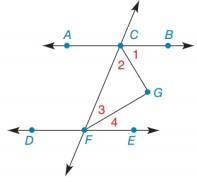Chapter 2.4, Problem 48E### Elementary Geometry for College St...

6th Edition
Daniel C. Alexander + 1 other
ISBN: 9781285195698

#### Solutions

Chapter
Section### Elementary Geometry for College St...

6th Edition
Daniel C. Alexander + 1 other
ISBN: 9781285195698
Textbook Problem
11 views

# Given: A B ↔ , D E ↔ and C F ↔ A B ↔ ∥ D E ↔ C G → bisects ∠ B C F F G → bisects ∠ C F EProve: ∠ G is a right angle.

To determine

To prove:

G is a right angle.

Explanation

Given:

AB, DE and CF

ABDE

CG bisects BCF

FG bisects CFE

Properties:

The sum of the three angles in a triangle is 180°.

Angle bisector bisects the angle in half.

Definition:

If two parallel lines are cut by a transversal, then the angles on the transversal side are called supplementary and the sum of the measures of the angles is 180°.

Calculation:

Consider the given figure.

Figure (1)

Calculate the sum of the angles of the given triangle.

mG+m2+m3=180°(1)

Since, the angle bisector bisect the angles in the half, then

m2=12(mBCF)m3=12(mCFE)</

### Still sussing out bartleby?

Check out a sample textbook solution.

See a sample solution

#### The Solution to Your Study Problems

Bartleby provides explanations to thousands of textbook problems written by our experts, many with advanced degrees!

Get Started